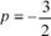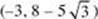﻿ ﻿CONIC SECTIONS - Coordinate Geometry - Geometry and Measurement - REVIEW OF MAJOR TOPICS - SAT SUBJECT TEST MATH LEVEL 2

## PART 2## REVIEW OF MAJOR TOPICS## CHAPTER 2 Geometry and Measurement

###2.1 Coordinate Geometry### CONIC SECTIONS

Geometrically, the conic sections are formed by the intersection of a plane and the two nappes of a right cone. The plane does not intersect the cone”s vertex (see below). In a parabola, the plane is parallel to a lateral edge of the cone. In a hyperbola, the plane is parallel to the axis of the cone. In an ellipse the plane is not parallel to either a lateral edge or the axis of the cone. Intersections that contain the cone”s vertex are called degenerate conics and are not covered on the Math Level 2 Test.

parabolahyperbolaellipseConics are also defined in terms of points in the coordinate plane. Formulas for the distance between two points and the distance between a point and a line are then used to derive standard equations of the conics. Each conic has a pair of standard equations, depending on whether the conic has an orientation to the x - or y-axis. With one exception described later, these are the only cases covered in the Math Level 2 Test.

Parabolas

A parabola is the set of points that are equidistant from a given point (focus) and a given line (directrix).

If a parabola has an x- orientation:

• It opens to the left or right.

• The standard equation is (yk)2 = 4p(xh).

• The focus is (h + p, k), and the directrix is x = hp.

• The vertex is (h, k).

• The common distance from the parabola to the focus and directrix is |p |.

• If p > 0, the parabola opens to the right; if p < 0, it opens to the left.

If a parabola has a y-orientation:

• It opens up or down.

• The standard equation is (xh)2 = 4p(yk).

• The focus is (h, k + p), and the directrix is y = kp.

• The vertex is (h, k).

• The common distance from the parabola to the focus and directrix is |p |.

• If p > 0, the parabola opens up; if p < 0, it opens down.

These points are illustrated in the figures below.

Graph of (y – k)2 = 4p(x – h) p > 0Graph of (x – h)2 = 4p(y – k) p < 0TIPWhen you studied quadratics in Algebra 2, x was the squared variable and the parabolas opened up or down. This should help you determine the correct orientation when you look at the equation.

Ellipses

An ellipse is the set of points whose distances from two given points (foci, plural of focus) sum to a constant.

If an ellipse has an x -orientation:

• The standard equation is, where a2 > b2.

• The major axis is parallel to the x -axis.

• The center is (h, k).

• The vertices are (ha, k) and (h + a, k), the endpoints of the major axis. So the major axis is 2a units long.

• The endpoints of the minor axis are (h, kb) and (h, k + b). So the minor axis is 2b units long.

• The foci are on the major axis. Each focus is at a distance offrom the center, so the foci are at (hc, k) and (h + c, k).

If an ellipse has a y -orientation:

• The standard equation is, where a2 > b2.

• The major axis is parallel to the y-axis.

• The center is (h, k).

• The vertices are (h, ka) and (h, k + a), the endpoints of the major axis.

• The endpoints of the minor axis are (hb, k) and (h + b, k).

• The foci are on the major axis. Each focus is at a distance offrom the center, so the foci are at (h, kc) and (h, k + c).

If the larger denominator in an equation of an ellipse is under the x-term, the ellipse has an x-orientation. If the larger denominator is under the y-term, the ellipse has a y-orientation.

Note that an ellipse with a = b is a circle whose equation is (xh)2 + (yk)2 = r2, where r is the common value of a and b .

These points are illustrated in the figures below.Hyperbolas

A hyperbola is the set of points whose distances from two fixed points (foci) differ by a constant. A hyperbola has two halves, corresponding to the two nappes of the cone. Each half has a vertex and sides that are asymptotic to a pair of intersecting lines.

If a hyperbola has an x-orientation:

• The hyperbola opens to the sides.

• The standard equation is.

• The center is (h, k).

• The vertices are (ha, k) and (h + a, k). The segment joining the two vertices is called the transverse axis. This axis is horizontal and has length 2a .

• The foci are on the transverse axis. The distance between the center and each focus is, so the foci are (hc, k) and (h + c, k).

• The vertical segment through the center with endpoints (h, kb) and (h, k + b), which has length 2b, is called the conjugate axis. The endpoints of this axis are not on the hyperbola.

• The equations of the asymptotes are.

If a hyperbola has a y-orientation:

• The hyperbola opens up and down.

• The standard equation is.

• The center is (h, k).

• The vertices are (h, ka) and (h, k + a). The transverse axis is vertical and has length 2a .

• The foci are (h, kc) and (h, k + c).

• The conjugate axis is horizontal, has endpoints (hb, k) and (h + b, k), and has length 2b .

• The equations of the asymptotes areIf the x-term of the equation is positive, the hyperbola has an x-orientation. If the y-term is positive, it has a y-orientation.

These points are illustrated in the figures below.The statement made earlier, that the only conic sections considered in the Math Level 2 test are those with axes parallel to the x - or y-axis, has one exception. The equation xy = k, where k is a constant, is the equation of a rectangular hyperbola where the asymptotes are the x - and y -axes. If k > 0, the branches of the hyperbola lie in the first and third quadrants. Otherwise, the branches lie in the second and fourth quadrants.

Eccentricity

The eccentricity of an ellipse or hyperbola is a measure of its degree of elongation. The eccentricity of an ellipse is, which is less than 1 since c < a . The closer c is to a, the more circular the ellipse. The eccentricity of a hyperbola is also. However, it is greater than 1 since c > a in a hyperbola. The farther c is from a, the more elongated the hyperbola.

EXAMPLES

1.Which conic section does this equation define? Also find, if they exist,

(i) the center

(ii) the vertex/vertices

(iii) the focus/foci

(iv) the directrix

(v) the asymptotes

(vi) the eccentricity

This is a hyperbola with a y -orientation. In this problem, h = 1, k = 3, a = 3, b = 4, and.

(i) The center is (1, 3).

(ii) The vertices are (1, 0) and (1, 6).

(iii) The foci are (1, –2) and (1, 8).

(iv) A hyperbola does not have a directrix.

(v) The asymptotes are.

(vi) The eccentricity is.

2. (y + 4)2 = –6(x – 2)

Which conic section does this equation define? Also find, if they exist,

(i) the center

(ii) the vertex/vertices

(iii) the focus/foci

(iv) the directrix

(v) the asymptotes

(vi) the eccentricity

This is a parabola with an x -orientation opening left. In this problem, h = 2, k = –4, and.

(i) A parabola does not have a center.

(ii) The vertex is (2, –4).

(iii) The focus is.

(iv) The directrix is.

(v) A parabola does not have asymptotes.

(vi) A parabola does not have eccentricity.

3.Which conic section does this equation define? Also find, if they exist,

(i) the center

(ii) the vertex/vertices

(iii) the focus/foci

(iv) the directrix

(v) the asymptotes

(vi) the eccentricity

This is an ellipse with a y-orientation since the larger denominator has y in the numerator. In this problem h = –3, k = 8, a = 10, b = 5, and.

(i) The center is (–3, 8).

(ii) The vertices are (–3, –2) and (–3, 18).

(iii) The foci areand.

(iv) An ellipse does not have a directrix.

(v) An ellipse does not have asymptotes.

(vi) The eccentricity is.

If an equation of a conic is not in standard form, completing the square will yield a standard-form equation. If the equation has squared terms in both x and y, completing the square in both variables will result in the standard equation of an ellipse or hyperbola. If only one of the variables is squared in the original equation, completing the square in that variable will result in the standard equation of a parabola.

4. Name the conic by finding its standard-form equation.

2x2 + 3y2 + 12x – 24y + 60 = 0This conic is an ellipse.

5. Name the conic by finding its standard-form equation.

4x2 + 4y2 – 12x – 20y – 2 = 0This is a circle with centerand radius 3.

6. Find the foci of the conic y2 + 2x + 2y = 5.

Since there is only one squared term, the conic is a parabola. A parabola has only one focus, (h + p, k) . Complete the square in y to get the standard equation:

(y + 1)2 = −2(x − 3)

Therefore, h = 3, k = −1, and p =.

The focus is.

7. Find the standard equation of a hyperbola with center (3, –4), vertices (3, 1) and (3, 7), and asymptotes.

Since the vertices have the same x-coordinate, the hyperbola has a y-orientation. The length of the transverse axis is 6, implying that a = 3. The slope of the asymptote is, so b = 4. The center is (h, k), so h = 3 and k = –4.

The standard equation is.

EXERCISES

1. Which of the following is a focus of(A) (1, –1)

(B) (2, –1)

(C) (3, –1)

(D) (2, –2)

(E) (–2, 1)

2. Which of the following is an asymptote of 3x2 – 4y2 – 12 = 0?

(A)(B)(C)(D)(E)3. The standard equation of a parabola with focus (2, –3) and directrix x = 6 is

(A) x – 2 = 8(y + 3)2

(B) x – 4 = –8(y + 3)2

(C) y + 3 = 8(x – 2)2

(D) y – 3 = –8(x + 2)2

(E) y – 3 = –8(x + 4)2

4. The standard equation of an ellipse with vertices (–5, 2) and (3, 2) and minor axis of length 6 is

(A)(B)(C)(D)(E)5. Which of the following is a vertex of 16x2y2 – 32x – 6y – 57 = 0?

(A) (1, –1)

(B) (1, 3)

(C) (1, 5)

(D) (–1, –3)

(E) (–1, 3)

6. The graph of x2 = (2y + 3)2 is

(A) an ellipse

(B) a parabola

(C) a hyperbola

(D) a circle

(E) none of these

﻿